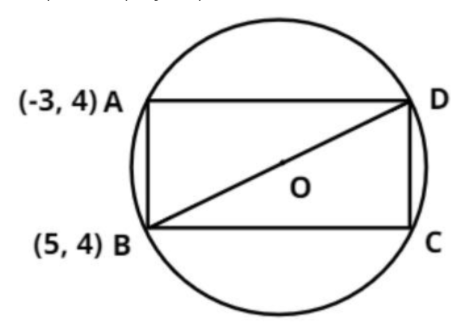Courses
Courses for Kids
Free study material
Free LIVE classes
MoreLIVE
Join Vedantu’s FREE Mastercalss

# One of the diameters of the circle circumscribing the rectangle ABCD is 4y = x+7. If A and B are the points (-3, 4) and (5, 4) respectively, then the area of the rectangle is  (a)${\text{ 21}}$  (b)${\text{ 32}}$  (c)${\text{ 35}}$  (d)${\text{ None of these}}$Verified
360k+ views

Hint – In this question it is given that the circle circumscribe the rectangle ABCD. Two side coordinates of the rectangle and equation of diameter is also given. So use the general equation of circle which is${\left( {x - g} \right)^2} + {\left( {y - f} \right)^2} = {r^2}$, and satisfy the points of the rectangle as it must lie on circle as well. Using the diameter and above concept, take out the radius and center of the circle. Then the length of diagonal could be taken out. Use Pythagoras to find out the length and breadth and thus eventually area.Let the general equation of the circle is

${\left( {x - g} \right)^2} + {\left( {y - f} \right)^2} = {r^2}$

Where (g, f) and r is the center and radius of the circle respectively.

Now it is given a circle circumscribing the rectangle ABCD (see figure).

Thus ABCD points touch the circle.

It is given that the coordinates of A = (-3, 4) and the coordinates of B = (5, 4)

So, these two points satisfy the equation of the circle.

$\Rightarrow {\left( { - 3 - g} \right)^2} + {\left( {4 - f} \right)^2} = {r^2}$……………………….. (1)

And, $\Rightarrow {\left( {5 - g} \right)^2} + {\left( {4 - f} \right)^2} = {r^2}$………………………. (2)

Now subtract equation (2) from equation (1) we have,

$\Rightarrow {\left( { - 3 - g} \right)^2} + {\left( {4 - f} \right)^2} - {\left( {5 - g} \right)^2} - {\left( {4 - f} \right)^2} = {r^2} - {r^2}$

$\Rightarrow {\left( { - 3 - g} \right)^2} - {\left( {5 - g} \right)^2} = 0$

Now simplify the above equation we have,

$\Rightarrow 9 + {g^2} + 6g - 25 - {g^2} + 10g = 0$

$\Rightarrow 16g = 16$

$\Rightarrow g = 1$

Now it is given that one of the diameter of the circle is $4y = x + 7$.

So, it passes through the center of the circle so it satisfies the point (g, f)

$\Rightarrow 4f = g + 7$

Now substitute the value of g in above equation we have,

$\Rightarrow 4f = 1 + 7 = 8$

$\Rightarrow f = \dfrac{8}{4} = 2$

So, the center of the circle is (1, 2).

Now from equation (1) calculate the radius of the circle we have,

$\Rightarrow {\left( { - 3 - 1} \right)^2} + {\left( {4 - 2} \right)^2} = {r^2}$

$\Rightarrow {r^2} = 16 + 4 = 20$

$\Rightarrow r = \sqrt {20} = 2\sqrt 5$

So, the diameter of the circle is $= 2r = 2 \times 2\sqrt 5 = 4\sqrt 5$.

And the diameter is the diagonal of the rectangle.

$\Rightarrow BD = 4\sqrt 5$

Now calculate the distance AB.

As we know that the distance between two points is given as

$d = \sqrt {{{\left( {{x_2} - {x_1}} \right)}^2} + {{\left( {{y_2} - {y_1}} \right)}^2}}$

The coordinates of A = (-3, 4) and the coordinates of B = (5, 4).

So, the distance AB = $\sqrt {{{\left( {5 - \left( { - 3} \right)} \right)}^2} + {{\left( {4 - 4} \right)}^2}}$

$\Rightarrow AB = \sqrt {{8^2}} = 8$

Now in the triangle ABD apply Pythagoras Theorem we have,

${\left( {{\text{Hypotenuse}}} \right)^2} = {\left( {{\text{Perpendicular}}} \right)^2} + {\left( {{\text{Base}}} \right)^2}$

$\Rightarrow {\left( {BD} \right)^2} = {\left( {AB} \right)^2} + {\left( {AD} \right)^2}$

$\Rightarrow {\left( {AD} \right)^2} = {\left( {4\sqrt 5 } \right)^2} - {\left( 8 \right)^2}$

$\Rightarrow {\left( {AD} \right)^2} = 80 - 64 = 16$

$\Rightarrow AD = \sqrt {16} = 4$

Now as we know that the area (A) of the rectangle is length (AB) multiplied by breadth (AD).

$\Rightarrow A = 8 \times 4 = 32$ Square unit.

Hence, option (b) is correct.

Note – Whenever we face such types of questions the key concept here is to have a good understanding of the general equation of circle. The diagrammatic representation of the information provided helps in better understanding of the geometry involved to help getting on the right track to reach the solution.

Last updated date: 20th Sep 2023
Total views: 360k
Views today: 3.60k